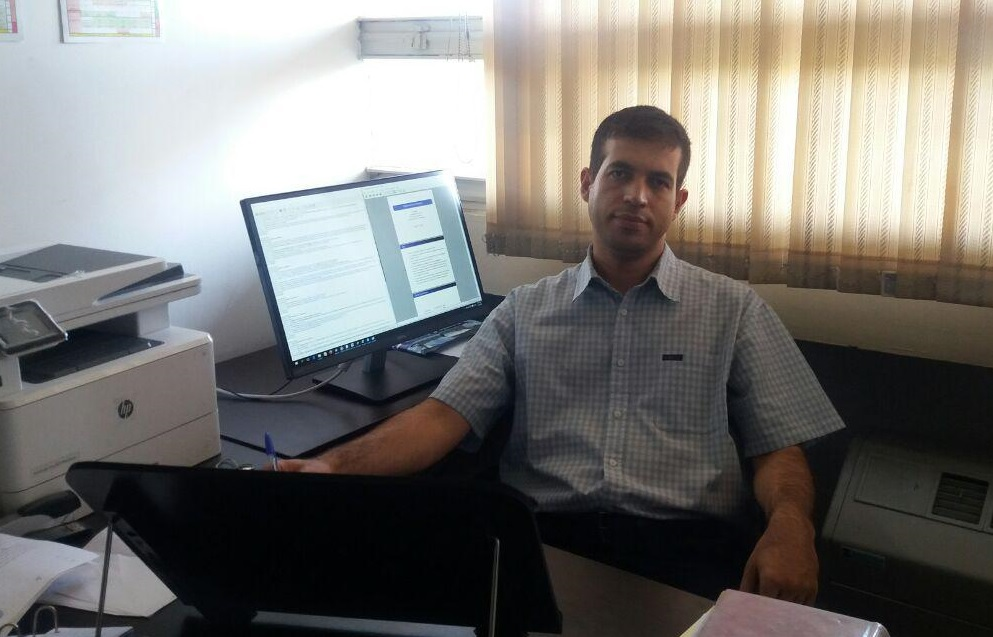Ali M. NassimiAssistant professor

Chemistry department

Sharif University of technology

Tehran, Iran

Phone: +98-21-66165377

Email: a.nassimi@sharif.edu

Room: 9-416

Education:

PhD, University of Toronto, 2011

M.Sc., Sharif University of Technology, 2006

B.Sc., Sharif University of Technology, 2004

Teaching:

Research in Theoretical chemistry:

Our group uses mathematics, data and computers to address chemical problems. Currently our group is focused on three specific problems.

Upper flammability limit is the greatest fuel concentration in a fuel-air gaseous mixture which enables self-propagation of a flame. Lower flammability limit (LFL) is the least fuel concentration in a fuel-air gaseous mixture which enables self-propagation of a flame. We have worked on using thermal theory of flame propagation for evaluating flammability limits and suggested density theory of flame propagation for evaluating flammability limits. Also we are combining thermal theory and density theory with a group contribution method for predicting flammability limits.

We know that chemical systems are governed by quantum mechanics and that the time evolution of a quantum system is given by the quantum Liouville equationwhere  and , respectively, are Hamiltonian operator and density matrix operator. The complexity of this equation scales exponentially with size of the system under consideration. Classical dynamics is given by classical-Liouville equationwhere H is the classical Hamiltonian and f is the phase space distribution function. Classical mechanics scales linearly with the size of the system and also is a first approximation to the exact quantum dynamics. To reduce the complexity while keeping the dynamics accurate one may use quantum-classical dynamicswhere  and  are functions of phase space variables regarding classical degrees of freedom and at the same time operators in the Hilbert space of quantum degrees of freedom. We are working on solving this equation in a mapping bases.

Dependence of non-redox reaction rate on the external electric field strength: We are developing a statistical mechanical model to predict the dependence of reaction rate constants on strength of external electric field.

ORCID identifier: 0000-0002-2639-0962